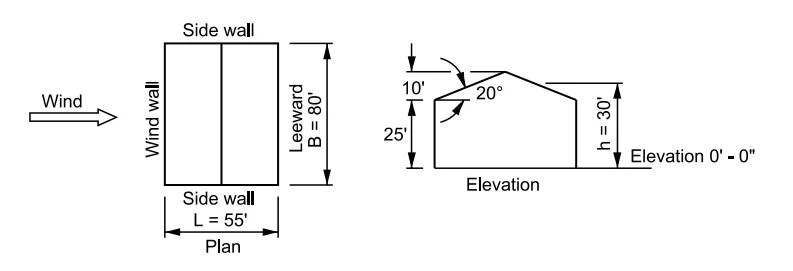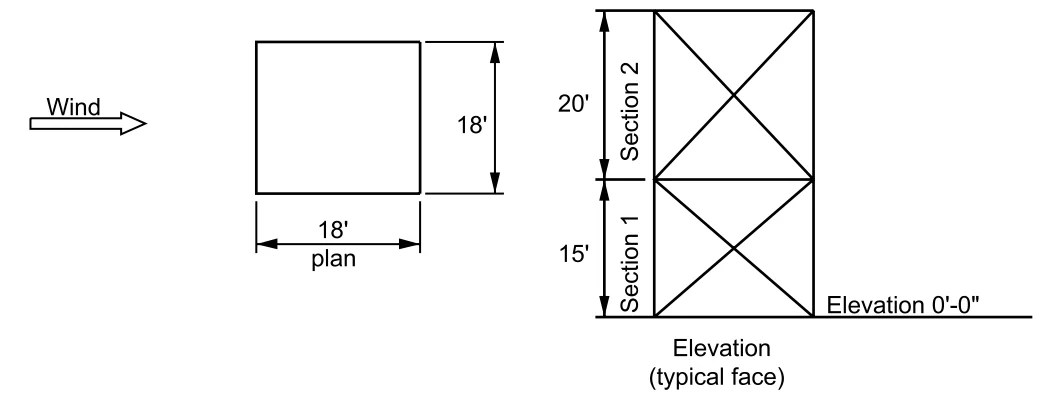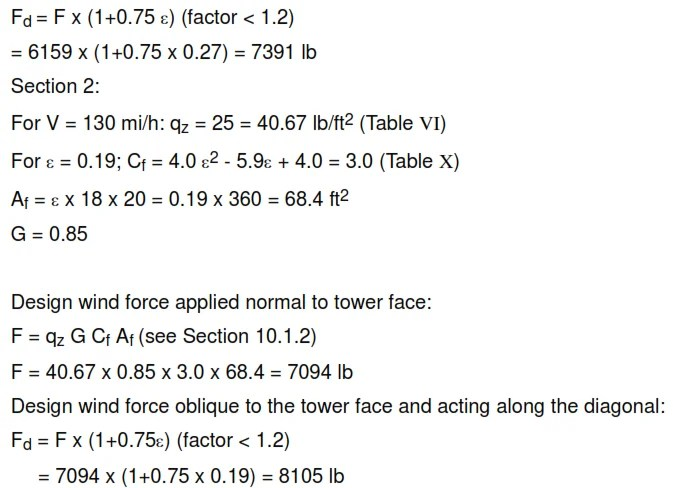Example 1: Determine the wind loading on the main wind force resisting frame for an enclosed building. See Figure 3 below. For this example, the assumed basic wind velocity is 115 mi/h, exposure C.
Note: For design wind pressure for all walls and roof, see 9.1.2. Imperial units are used to illustrate the examples only.

Figure 3 – Building Description – Example 1V (basic wind velocity) = 115 mi/h; exposure C
Windward wall
For V = 115 mi/h:Side walls
Cp = -0.7 (Table VIII)Note: Leeward wall wind loading is always uniform for any building height.

Example 2: Determine the wind loading on the main wind-force resisting frame for a square-section trussed tower (Ɛ = 0.27 for Section 1; Ɛ = 0.19 for Section 2). See Figure 5 below. For this example, the assumed wind velocity is 130 mi/h, exposure C.

Figure 5 – Diagram Description – Example 2Figure 5 – Diagram Description – Example 2

V (basic wind velocity) = 130 mi/h; exposure C.

Section 1:Design wind force oblique to the tower face and acting along the diagonal:### Information about wind-force resisting system

Structures sensitive to wind, for example tall buildings with a height-to-width ratio greater than 5, stacks, and process columns shall be analyzed in accordance with ASCE 7-95 (methods specified in Table 6-1 and paragraph 6.6 of the commentary), or by a consultant recognized as being knowledgeable in this field. A rational analysis that incorporates the dynamic properties of the main wind-force resisting system is often needed to determine the response of these structures to the wind.

The following are changes to ASCE 7-95 with respect to ASCE 7-93.

a. Revised wind speed (V) based on 3-second gust speed.

b. Revised velocity pressure (qz) formulation.

c. Revised velocity pressure exposure coefficient (Kz). Revised gust response factor (G). Revised importance factor (I).

f. Revised classification categories of buildings and other structures.

paktechpoint.com

#### You may also like...

error: tected !!Content is pro